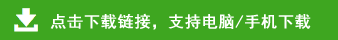# 小学数学三年级口算+应用题练习大全

370－190=   1900－540=   10×18=   45×19=
920÷4=   60－12=   2300－800=   80×0=
53×21=   90÷6=   85－50=   274－45=
40×70=   7248÷4=   48÷16=   66－6=
355－29=   30×20=   96÷6=   96÷24=
1000－20=   476×8＝   50×60=   3560-12=
900÷45=   640－40=   1040×5＝   21×5=
24375÷5=   6240÷20=